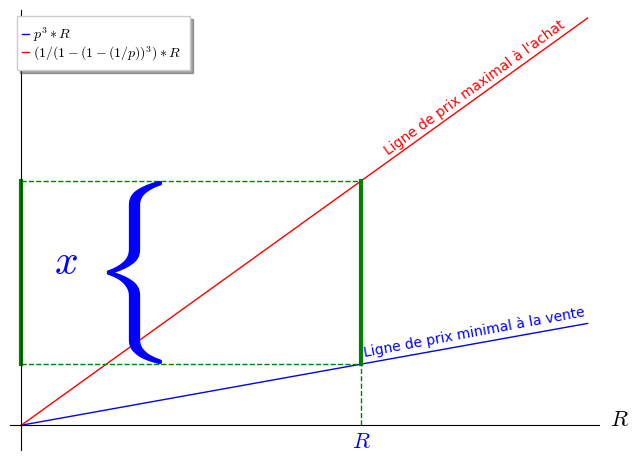# Revision history [back]

Here is a version with the brace at the right location.

Is that what you want or is that what you would call ugly?

Maybe you want a thinner brace?

sage: var('R')
sage: p = 1/2
sage: pp = p^3
sage: qq = 1/(1-(1-(1/p))^3)
sage: pp6 = pp*6
sage: qq6 = qq*6
sage: opt = {'ticks': [[], []], 'axes_labels': ['$R$', '']}
sage: g = plot(p^3*R, (R, 0, 10), legend_label='$p^3*R$', **opt)
sage: g += plot(qq*R, (R, 0, 10), color="red", legend_label='$(1/(1-(1-(1/p))^3)*R$', **opt)
sage: g += line([(6, pp6), (6, qq6)], color="green", thickness=3, **opt)
sage: g += line([(0, pp6), (0, qq6)], color="green", thickness=3, **opt)
sage: g += line([(6, 0), (6, pp6)], color="green", linestyle="--", **opt)
sage: g += line([(0, pp6), (6, pp6)], color="green", linestyle="--", **opt)
sage: g += line([(0, qq6), (6, qq6)], color="green", linestyle="--", **opt)
sage: g += text("Ligne de prix maximal à l'achat", (8, 4.15), rotation=36.0, color="red")
sage: g += text("Ligne de prix minimal à la vente", (8, 1.15), rotation=10.5, color="blue")
sage: g += text(r"$\left\{\right.$", (2.5, 1.515), fontsize=110, horizontal_alignment='right')
sage: g += text("$R$", (6, -.2), fontsize=16)
sage: g += text("$x$", (1, 2), fontsize=30, horizontal_alignment='right')
sage: show(g, **opt)